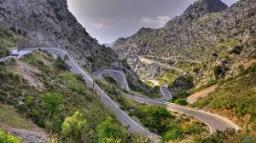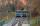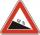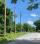# Height difference

What height difference is overcome if we pass a road 1 km long with a pitch21 per mille?

x =  20.9954 m

### Step-by-step explanation:Did you find an error or inaccuracy? Feel free to write us. Thank you!Tips to related online calculators

#### You need to know the following knowledge to solve this word math problem:

We encourage you to watch this tutorial video on this math problem:

## Related math problems and questions:Between cities A and B is route 13 km long of stúpanie average 7‰. Calculate the height difference of cities A and B.
• RailwayRailway line had on 5.8 km segment climb 9 permille. How many meters track ascent?The average climb of the road is given by ratio 1:15. By what angle road average climb?
• ClimbOn the road sign, which informs the climb is 8.7%. The car drive 5 km along this road. What is the height difference that the car went to?
• ClimbFor horizontal distance 4.2 km road rise by 6.3 m. Calculate the road pitch in ‰ (permille, parts per thousand).
• ClimbRoad has climbing 1:27. How big is a angle corresponds to this climbing?On a straight stretch of road is marked 12 percent drop. What angle makes the direction of the road with the horizontal plane?The angle of a straight road is approximately 12 degrees. Determine the percentage of this road.
• SteepleWe see the church tower from the road at an angle of 52°. When we zoom out to 29 meters away, it can be seen at an angle of 21°. How high is it?
• CablecarFunicular on Petrin (Prague) was 408 meters long and overcomes the difference 106 meters in altitude. Calculate the angle of climb.Road sign informs the gradient is 10.9%. Calculate the angle which average decreases.What angle rising stairway if step height in 20 cm and width 26 cm?I have bevel in the ratio 1:6. What is the angle and how do I calculate it?There is a traffic sign for climbing on the road with an angle of 7%. Calculate at what angle the road rises (falls).Calculate the average slope (in permille and even in degrees) of the rail tracks between Prievidza (309 m AMSL) and Nitrianske Pravno (354 m AMSL), if the track is 11 km long.Side b = 1.5, hypotenuse angle A = 70 degrees, Angle B = 20 degrees. Find its unknown sides length.On a straight road, three pillars are 6 m high at the same distance of 10 m. At what angle of view does Vlado see each pillar if it is 30 m from the first and his eyes are at 1.8 m high?